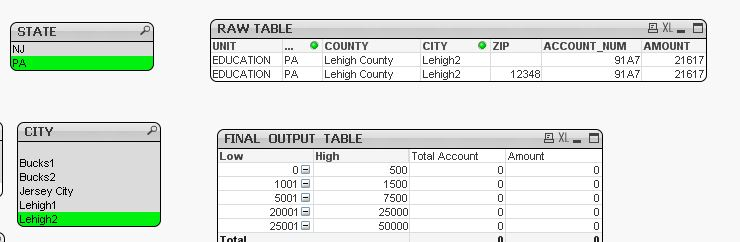# New to QlikView

Discussion board where members can get started with QlikView.

Highlighted
Contributor

## How to sum values based on selections at different levels

Hello,

Below is my raw table and final output table with bands (range). However, my expressions (Total Account & Amount) aren't working.

Attached is a sample qvw.

Based on what selection (State, County, City or Zip) is made, the Amount should fall in the corresponding range.
Selection can be one state or multiple states and so forth. I tried using set analysis but didn't quite get the correct result.

Any ideas how this can be handled? Thanks!1 Solution

Accepted SolutionsMVP

## Re: How to sum values based on selections at different levels

Try this

Sum({<ZIP = {"=If(GetSelectedCount(ZIP) > 0, Len(Trim(ZIP)) > 0, Len(Trim(ZIP)) = 0)"},

COUNTY = {"=If(GetSelectedCount(COUNTY) > 0 or GetSelectedCount(CITY) > 0 or GetSelectedCount(ZIP) > 0, Len(Trim(COUNTY)) > 0, Len(Trim(COUNTY)) = 0)"},

CITY = {"=If(GetSelectedCount(CITY) > 0 or GetSelectedCount(ZIP) > 0, Len(Trim(CITY)) > 0, Len(Trim(CITY)) = 0)"}>}AMOUNT) + Sum({1} 0)

6 RepliesMVP

## Re: How to sum values based on selections at different levels

What is the expected output for the data provided?

Contributor

## Re: How to sum values based on selections at different levels

Hi Sunny, please find the attached excel file for the expected output.MVP

## Re: How to sum values based on selections at different levels

This expression almost works... see if you have missed some data rows and it works for your original app

Sum({<ZIP = {"=If(GetSelectedCount(ZIP) > 0, Len(Trim(ZIP)) > 0, Len(Trim(ZIP)) = 0)"}, COUNTY = {"=If(GetSelectedCount(COUNTY) > 0, Len(Trim(COUNTY)) > 0, Len(Trim(COUNTY)) = 0)"}, CITY = {"=If(GetSelectedCount(CITY) > 0, Len(Trim(CITY)) > 0, Len(Trim(CITY)) = 0)"}>}AMOUNT) + Sum({1} 0)

Count(DISTINCT {<ZIP = {"=If(GetSelectedCount(ZIP) > 0, Len(Trim(ZIP)) > 0, Len(Trim(ZIP)) = 0)"}, COUNTY = {"=If(GetSelectedCount(COUNTY) > 0, Len(Trim(COUNTY)) > 0, Len(Trim(COUNTY)) = 0)"}, CITY = {"=If(GetSelectedCount(CITY) > 0, Len(Trim(CITY)) > 0, Len(Trim(CITY)) = 0)"}>}ACCOUNT_NUM) + Sum({1} 0)

Contributor

## Re: How to sum values based on selections at different levelsHi Sunny, yes you are right - I think I missed some data rows. However, during my testing I found this expression didn't work in the above examples. Screen shots attached.

1) First image, when NJ state and Lehigh2 city is selected, the final table displays 0. Correct total account is 1 and amount is 21617
2) Second image, when NJ state and Lehigh1+Lehigh2 city is selected, the final table still displays 0. Correct total account is 2 and amount is 21617 + 1125 = 22742

Contributor

## Re: How to sum values based on selections at different levels

I meant state = PA (not NJ) in my prior response. Correction.MVP

## Re: How to sum values based on selections at different levels

Try this

Sum({<ZIP = {"=If(GetSelectedCount(ZIP) > 0, Len(Trim(ZIP)) > 0, Len(Trim(ZIP)) = 0)"},

COUNTY = {"=If(GetSelectedCount(COUNTY) > 0 or GetSelectedCount(CITY) > 0 or GetSelectedCount(ZIP) > 0, Len(Trim(COUNTY)) > 0, Len(Trim(COUNTY)) = 0)"},

CITY = {"=If(GetSelectedCount(CITY) > 0 or GetSelectedCount(ZIP) > 0, Len(Trim(CITY)) > 0, Len(Trim(CITY)) = 0)"}>}AMOUNT) + Sum({1} 0)